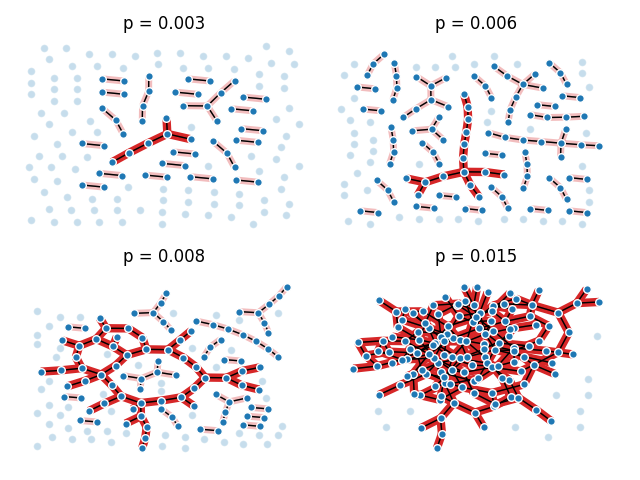# 巨型构件¶#    Copyright (C) 2006-2019
#    Aric Hagberg <hagberg@lanl.gov>
#    Dan Schult <dschult@colgate.edu>
#    Pieter Swart <swart@lanl.gov>

import math

import matplotlib.pyplot as plt
import networkx as nx

try:
import pygraphviz
from networkx.drawing.nx_agraph import graphviz_layout
layout = graphviz_layout
except ImportError:
try:
import pydot
from networkx.drawing.nx_pydot import graphviz_layout
layout = graphviz_layout
except ImportError:
"drawing with spring layout;\n"
"will be slow.")
layout = nx.spring_layout

n = 150  # 150 nodes
# p value at which giant component (of size log(n) nodes) is expected
p_giant = 1.0 / (n - 1)
# p value at which graph is expected to become completely connected
p_conn = math.log(n) / float(n)

# the following range of p values should be close to the threshold
pvals = [0.003, 0.006, 0.008, 0.015]

region = 220  # for pylab 2x2 subplot layout
plt.subplots_adjust(left=0, right=1, bottom=0, top=0.95, wspace=0.01, hspace=0.01)
for p in pvals:
G = nx.binomial_graph(n, p)
pos = layout(G)
region += 1
plt.subplot(region)
plt.title("p = %6.3f" % (p))
nx.draw(G, pos,
with_labels=False,
node_size=10
)
# identify largest connected component
Gcc = sorted(nx.connected_components(G), key=len, reverse=True)
G0 = G.subgraph(Gcc)
nx.draw_networkx_edges(G0, pos,
with_labels=False,
edge_color='r',
width=6.0
)
# show other connected components
for Gi in Gcc[1:]:
if len(Gi) > 1:
nx.draw_networkx_edges(G.subgraph(Gi), pos,
with_labels=False,
edge_color='r',
alpha=0.3,
width=5.0
)
plt.show()


Total running time of the script: ( 0 minutes 0.814 seconds)

Gallery generated by Sphinx-Gallery